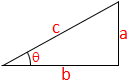# Arctan Calculator

In mathematics, the inverse trigonometric functions are the inverse functions of the trigonometric functions. Specifically, the arctan is the inverse of the tangent. It is normally represented by arctan(θ) or tan-1(θ).arctan = ?